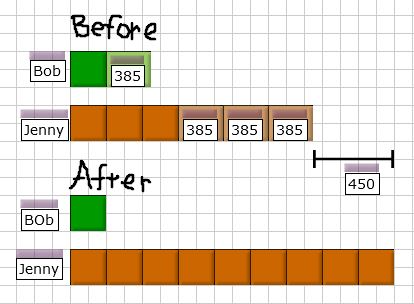# Question

Hi, can help with this qn? Thanks!

______

The ratio of the amount of money Bob had to the amount of money Jenny had was 1:3. After Bob spent  \$385 and Jenny received \$450 from her father, Jenny had 9 times as much money as Bob. How much money did Jenny have at first?

**we got a different answer front the one given.

2 Answers

# Answer

At first —
Bob : 1 unit
Jenny : 3 units

After —
Bob : 1 unit – 385
Jenny : 3 units + 450

(1 unit – 385) x 9 = 9 units – 3465 ——- 3 units + 450
1 unit = (450 + 3465)/(9 – 3) = 652.50
3 units = 1957.50

Ans : \$1957.50.

0 Replies 0 Likes6 units –> 3 x \$385 + \$450 = \$1605

3 units –> \$1605 / 2 = \$802.50

Jenny (Before) –> \$802.50 + 3 x \$385 = \$1957.50

Jenny had \$1957.50 at first.

0 Replies 0 Likes